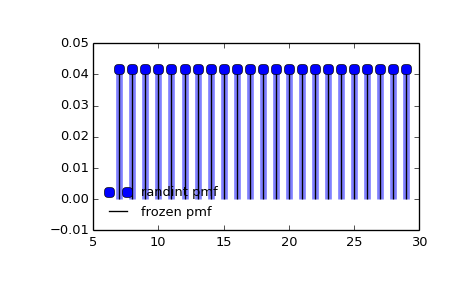# scipy.stats.randint¶

scipy.stats.randint = <scipy.stats._discrete_distns.randint_gen object at 0x450c038c>[source]

A uniform discrete random variable.

As an instance of the rv_discrete class, randint object inherits from it a collection of generic methods (see below for the full list), and completes them with details specific for this particular distribution.

Notes

The probability mass function for randint is:

randint.pmf(k) = 1./(high - low)


for k = low, ..., high - 1.

randint takes low and high as shape parameters.

Note the difference to the numpy random_integers which returns integers on a closed interval [low, high].

The probability mass function above is defined in the “standardized” form. To shift distribution use the loc parameter. Specifically, randint.pmf(k, low, high, loc) is identically equivalent to randint.pmf(k - loc, low, high).

Examples

>>> from scipy.stats import randint
>>> import matplotlib.pyplot as plt
>>> fig, ax = plt.subplots(1, 1)


Calculate a few first moments:

>>> low, high = 7, 31
>>> mean, var, skew, kurt = randint.stats(low, high, moments='mvsk')


Display the probability mass function (pmf):

>>> x = np.arange(randint.ppf(0.01, low, high),
...               randint.ppf(0.99, low, high))
>>> ax.plot(x, randint.pmf(x, low, high), 'bo', ms=8, label='randint pmf')
>>> ax.vlines(x, 0, randint.pmf(x, low, high), colors='b', lw=5, alpha=0.5)


Alternatively, the distribution object can be called (as a function) to fix the shape and location. This returns a “frozen” RV object holding the given parameters fixed.

Freeze the distribution and display the frozen pmf:

>>> rv = randint(low, high)
>>> ax.vlines(x, 0, rv.pmf(x), colors='k', linestyles='-', lw=1,
...         label='frozen pmf')
>>> ax.legend(loc='best', frameon=False)
>>> plt.show()Check accuracy of cdf and ppf:

>>> prob = randint.cdf(x, low, high)
>>> np.allclose(x, randint.ppf(prob, low, high))
True


Generate random numbers:

>>> r = randint.rvs(low, high, size=1000)


Methods

 rvs(low, high, loc=0, size=1, random_state=None) Random variates. pmf(x, low, high, loc=0) Probability mass function. logpmf(x, low, high, loc=0) Log of the probability mass function. cdf(x, low, high, loc=0) Cumulative density function. logcdf(x, low, high, loc=0) Log of the cumulative density function. sf(x, low, high, loc=0) Survival function (1 - cdf — sometimes more accurate). logsf(x, low, high, loc=0) Log of the survival function. ppf(q, low, high, loc=0) Percent point function (inverse of cdf — percentiles). isf(q, low, high, loc=0) Inverse survival function (inverse of sf). stats(low, high, loc=0, moments='mv') Mean(‘m’), variance(‘v’), skew(‘s’), and/or kurtosis(‘k’). entropy(low, high, loc=0) (Differential) entropy of the RV. expect(func, low, high, loc=0, lb=None, ub=None, conditional=False) Expected value of a function (of one argument) with respect to the distribution. median(low, high, loc=0) Median of the distribution. mean(low, high, loc=0) Mean of the distribution. var(low, high, loc=0) Variance of the distribution. std(low, high, loc=0) Standard deviation of the distribution. interval(alpha, low, high, loc=0) Endpoints of the range that contains alpha percent of the distribution

#### Previous topic

scipy.stats.poisson

#### Next topic

scipy.stats.skellam